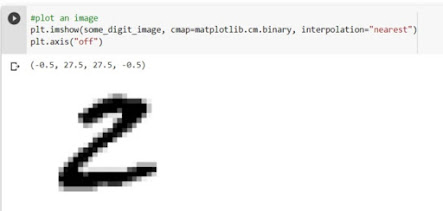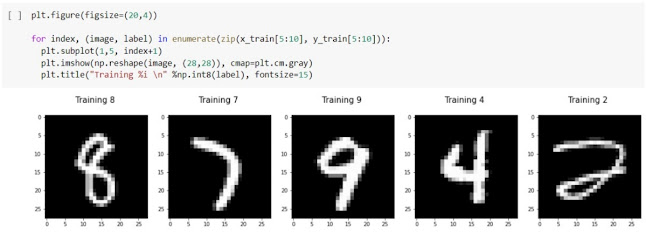# HandWritten Digit Recognition on MNIST Dataset | Machine Learning Project using Python

#### Hello and welcome,

Machine learning problems are about learning from the data to predict the "Behaviour" of unknown data or upcoming data. The digits example is a classic and it's like a hello world! in ML.Handwritten Digit Recognition

In this blog, we are going to solve this problem from scratch by installing all the tools, understanding the code, and using a very well-known library called scikit-learn. Let's get started.

You can use Jupyter Notebook or Google Colab for developing the project. I will use Goggle Colab and will make the notebook available for you in case any doubt arises.

## Project Overview

There are some scanned images(handwritten digits) in the dataset and for each digit, we know the label(digit) it represents. for instance, there's an image, we know it's representing number 7. the problem statement is to make the ML model capable to learn from all images and predict the correct outcome on unseen images or digits.

## Dataset Overview

Sklearn has some built-in datasets that allow you to start quickly without downloading any external datasets. If you want to download any external dataset of digits you can. I am going to use a popular Sklearn dataset known as the MNIST dataset.

``````from sklearn.datasets import fetch_openml
mnist = fetch_openml('mnist_784')
``````

Now, to see the data and target variable use the following code.

``````print(mnist.data.shape)
print(mnist.target.shape)
``````

when you will run both statements, you will see that the data consist of 70000 rows and 70000 labels. Now what we will do is take the data and target features in different variables to have a cleaned analysis.

x, y = mnist['data'], mnist['target']

when you will run or try to see the x, what are you seeing in that output?  it is a one-dimensional data or array. so, the dataset contains the images in form of pixels in which rows are stacked to form a digit in form of an array. so, the dataset consists of 28 by 28 grayscale images.

GrayScale Image: Grayscale image is simply one in which the only colors are a shade of gray(composed exclusively of shades of gray). The reason for differentiating such images from any sort of color image is that less information needs to be provided for each pixel.

### Sample digit into an image

To see this array in form of an image we need to reshape it into 28*28. for plotting the image we will be using a popular visualization library known as Matplotlib.

``````import matplotlib
import matplotlib.pyplot as plt
``````

I will show an example of 1 label by plotting, you can try with a different label too.

``````some_digit = x
some_digit_image = some_digit.reshape(28,28)

#Plot an image
plt.imshow(some_digit_image, cmap=matplotlib.cm.binary, interpolation="nearest")
plt.axis("off")
``````Digit to Image Visualization

If you will check the label of y then you will get 2 as output. means, the image we plotted is correctly labeled in a dataset.

## Splitting Dataset into Training and Testing Set

The MNIST dataset which we are using is pre-splitted into the training and testing set as the first 60000 data is training and the last 10000 data is a test set. so we will move with this only.
``````x_train, x_test = x[: 60000], x[60000 :]
y_train, y_test = y[: 60000], y[60000 :]
``````

If you want you can simply use a train test split with a test size of 1/7.0 and a random state of your choice to obtain a proper ratio of train and test set. here, I am going to randomly shuffle the train set to include each distribution, and as we have simply taken the train set so, to avoid any kind of noise that the model tries to learn.
``````import numpy as np
shuffle_index = np.random.permutation(60000)
x_train, y_train = x_train[shuffle_index], y_train[shuffle_index]
``````
Showing Images and LabelsPlotting Digits with labels

It is not a binary classification, we have to classify 0-9 digits. I will give one example in this by building a 2 detector in which we will implement this as a binary classification to see that is the entered digit is 2 or not, then we will implement the model on the complete dataset.

## Building a 2- Detector

``````y_train = y_train.astype(np.int8)
y_test = y_test.astype(np.int8)
y_train_2 = (y_train == 2)
y_test_2 = (y_test == 2)
``````

Now, implement a model. for binary classification we know the first model we test is logistic regression. so, let's import and train it.
``````from sklearn.linear_model import LogisticRegression
log_clf = LogisticRegression()

#Now train it.
log_clf.fit(x_train, y_train_2)

#Test it, on the sample digit we have plotted early because we know it's a label.
log_clf.predict([some_digit])
``````
If you get the output as true because in my case it's 2 only. so your model is performing correctly.
We can cross-validate it and know the accuracy score of our model.
``````from sklearn.model_selection import cross_val_score
cross_val_score(log_clf, x_train, y_train_2, scoring="accuracy")
``````

You will get the accuracy near about 95 to 97 percent. It is very nice accuracy but it's a binary classification, not let's go and implement it on the full dataset.
``````clf = LogicticRegression(solver="lbfgs")
clf.fit(x_train, y_train)

#Now, you can predict it on new data(new images)
clf.predict(x_test.reshape(-1, 1)

#check the label, is it correct to not
y_test
``````

Then, predict data on multiple observations and then on complete datasets.

## Measuring Model Performance

There are many metrics to measure the performance of a model but for ease of simplicity, I am moving with an accuracy score.
``````score = clf.score(x_test, y_test)
print(f"accuracy_score: {score}")
``````

The score is nice, we got an accuracy score of approximately 92 percent.

## Displaying the Misclassified Images with Predicted labels

Take the index from the dataset where our model has been classified wrong.
``````index = 0
misclassified_img = []
for label, predict in zip(y_test, predictions):
if label != predict:
misclassified_img.append(index)
index += 1
``````

#### Plot the graph of misclassified imagesPlotting Missclassified Images

Now, we have finished our project.

🎯 The Complete Notebook can be found 👉  Handwritten Digit Recognition

## CONCLUSION

I hope, you enjoyed the project here, The important thing is making a Machine learning model in Sklearn is not a lot of work. The main thing is to get the concepts that are running behind it. I hope that the blog is helpful for you to find the best from this and whatever your motive for the project is.

Thank you so much 😊

If you have any doubt or suggestions then, please let me know.

1.Keep the
Good
Work up...

2.Very nice detail explanation of each step. I like your colab notebook and code.

1.Thank You.

3.4.Good work 👍😁

5.Thank u for such valueable content ..